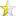Jul 15, 2008

# Recusion TypesIntroduction

Here I am going to give a detail about Recursion in C++. Definition: Recursion is the process where a function is called itself but stack frame will be out of limit because function call will be infinite times. So a termination condition is mandatory to a recursion.

Many complex problem can be solved by recursion in a simple code. But it's too much costly than iterative. because in every recursion call one stack frame will formed.You all already know that about it's cost. but if problem is very complex than no way to solve except recursion.

Background

First recursion came into mathematics and then came into Computer science. Idea of it's use that first broke your problem into subproblems and solve it by using recursion.

The code

In C++, Recursion can be divided into two types:
(a)Run- Time Recursion: Normal as in C
(b)Compile- Time Recursion: By using Template

Each of these can be also divided into following types:

1. Linear Recursion
2. Binary Recursion
3. Tail Recursion
4. Mutual Recursion
5. Nested Recursion

1. Linear Recursion: This recursion is the most commonly used. In this recursion a function call itself in a simple manner and by termination condition it terminates. This process called 'Winding' and when it returns to caller that is called 'Un-Winding'. Termination condition also known as Base condition.

Example: Factorial calculation by linear recursion

Run-Time Version

Code:

int Fact(long n)
{
if(0>n)
return -1;
if(0 == n)
return 1;
else
{
return ( n* Fact(n-1));
}
}

Winding Process:

Function called Function return

Fact(6) 6*Fact(5)
Fact(5) 5*Fact(4)
Fact(4) 4*Fact(3)
Fact(3) 3* Fact(2)
Fact(2) 2* Fact(1)
Fact(1) 1* Fact(0)
Terminating Point
Fact(0) 1

Unwinding Process

Fact(1) 1*1
Fact(2) 2*1
Fact(3) 3*2*1
Fact(4) 4*3*2*1
Fact(5) 5*4*3*2*1
Fact(6) 6*5*4*3*2*1

Compile-Time Version

Code:

// template for Base Condition
template <>
struct Fact<0>
{
enum
{
factVal = 1
};
};

template
struct Fact
{
// Recursion call by linear method
enum
{
value = n * Fact::factVal
};
};

To test it how it's working at compile time, just call
cout <<>::factVal ;
And compile it then compiler error will come, because no template for -1.

2. Binary Recursion: Binary Recursion is a process where function is called twice at a time inplace of once at a time. Mostly it's using in data structure like operations for tree as traversal, finding height, merging, etc.

Example: Fibonacci number

Run Time Version Code
Code:

int FibNum(int n)
{
// Base conditions
if (n < 1)
return -1;
if (1 == n || 2 == n)
return 1; // Recursive call by Binary Method
return FibNum(n - 1) + FibNum(n - 2);
// At a time two recursive function called so Binary
}
// binary }

Compile Time Version Code
Code:

// Base Conditions
template<>
struct FibNum<2>
{
enum { val = 1 };
};
template <>
struct FibNum<1>
{
enum { val = 1 };
};

// Recursive call by Binary Method
template
struct FibNum
{
enum { val= FibNum::val + FibNum::val };
};

3. Tail Recursion: In this method, recursive function is called at the last. So it's more efficient than linear recursion method. Means you can say termination point will come(100%) only you have to put that condition.

Example: Fibonacci number

Run Time Version Code
Code:

int FibNum(int n, int x, int y)
{
if (1 == n) // Base Condition
{
return y;
}
else // Recursive call by Tail method
{
return FibNum(n-1, y, x+y);
}
}

Compile Time Version Code

Code:

template
struct FibNum
{
// Recursive call By tail method
enum
{
val = FibNum::val
};
};

// Base Condition or Termination
template
struct FibNum<1,>
{
enum
{
val = y
};
};

4. Mutual Recursion: Functions calling each other. Let's say FunA calling FunB and FunB calling FunA recursively. This is not actually not recursive but it's doing same as recursive. So you can say Programming languages which are not supporting recursive calls, mutual recursion can be applied there to fulfill the requirement of recursion. Base condition can be applied to any into one or more than one or all functions.

Example: To find Even Or Odd number

Run Time Version Code
Code:

bool IsOddNumber(int n)
{
// Base or Termination Condition
if (0 == n)
return 0;
else
// Recursive call by Mutual Method
return IsEvenNumber(n - 1);
}
bool IsEvenNumber(int n)
{
// Base or Termination Condition
if (0 == n)
return 1;
else
// Recursive call by Mutual Method
return IsOddNumber(n - 1);
}

Compile Time Version Code
Code:

// Base Or Termination Conditions
template <>
struct IsOddNumber<0>
{
enum
{
val = 0
};
};
template <>
struct IsEvenNumber<0>
{
enum
{
val = 1
};
};

// Recursive calls by Mutual Method

template
struct IsOddNumber
{
enum
{
val = n == 0 ? 0 : IsEvenNumber::val
};
};

template
struct IsEvenNumber
{
enum
{
val = n == 0 ? 1 : IsOddNumber::val
};
};

5.Nested Recursion: It's very different than all recursions. All recursion can be converted to iterative (loop) except nested recursion. You can understand this recursion by example of Ackermann function.

Example: Ackermann function

Run Time Version Code
Code:

int Ackermann(int x, int y)
{
// Base or Termination Condition
if (0 == x)
{
return y + 1;
}
// Error Handling condition
if (x <> 0 && 0 == y)
{
return Ackermann(x-1, 1);
}
// Recursive call by Nested method
else
{
return Ackermann(x-1, Ackermann(x, y-1));
}
}

Compile Time Version Code
Code:

// Base Or Termination condition
template
struct Ackermann<0,>
{
enum { val = y + 1 };
};

// Recursive Call by Linear Method
template
struct Ackermann
{
enum
{
val = Ackermann::val
};
};
// Recursive Call by Nested Method
template
struct Ackermann
{
Enum
{
val = Ackermann ::val>::val
};
};